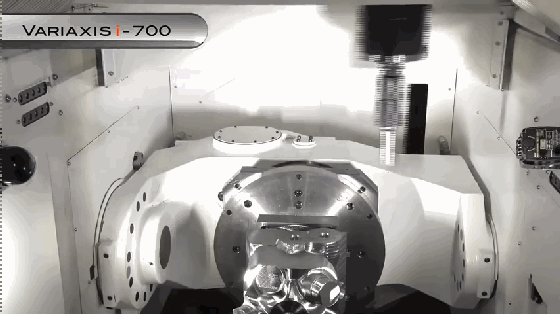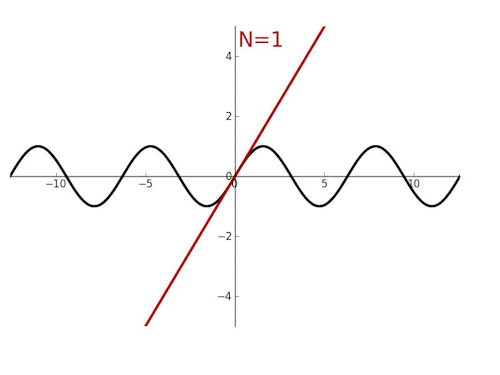# 关注数学发展,弘扬科学精神,专注数学科普

## 如何通俗理解泰勒公式？sinx~x/1!+x^3/3!+x^5/5!+⋯+x^n/n!，n为基数。

cosx-e^(-x^2/2)=[1-x^2/2!+x^4/4!+o(x^4 )]-[1-x^2/2+1/2!  x^4/4+o(x^4 )]=-1/12 x^4+o(x^4)

e=1+1/2!+1/3!+1/4!+⋯

（声明：本文仅代表作者观点，不代表本站观点，仅做陈列之用）

[责编：云峰]